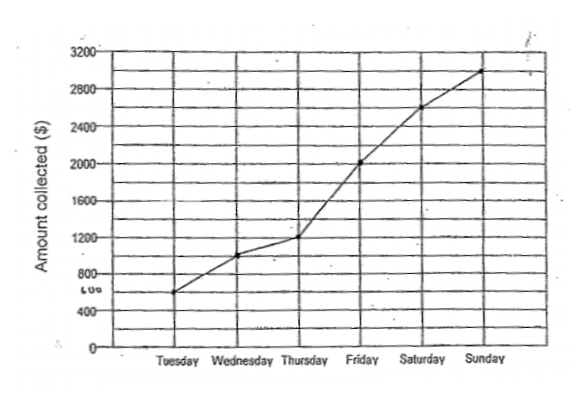# Question 1 of 29

The line graph below shows the amount of money collected during a donation drive from Tuesday to Sunday. Express the amount of money collected on Tuesday as a fraction of the amount of money collected on Saturday. Give your answer in its simplest form.A
$\frac{1}{9}$
B
$\frac{2}{11}$
C
$\frac{3}{13}$
D
$\frac{4}{18}$
E
None of the above